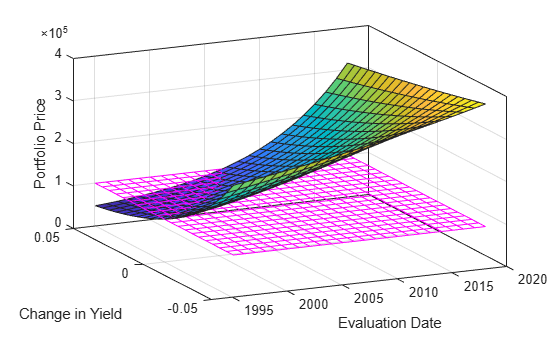# Bond Prices and Yield Curve Parallel Shifts

This example uses Financial Toolbox™ bond pricing functions to evaluate the impact of time-to-maturity and yield variation on the price of a bond portfolio. Also, this example shows how to visualize the price behavior of a portfolio of bonds over a wide range of yield curve scenarios, and as time progresses toward maturity.

Specify values for the settlement date, maturity date, face value, coupon rate, and coupon payment periodicity of a four-bond portfolio. For simplicity, accept default values for the end-of-month payment rule (rule in effect) and day-count basis (actual/actual). Also, synchronize the coupon payment structure to the maturity date (no odd first or last coupon dates). Any inputs for which defaults are accepted are set to empty matrices (`[]`) as placeholders where appropriate. Also, specify the points on the yield curve for each bond.

```Settle = datetime(1995,1,15); Maturity = datetime( [2020, 4, 3;... 2025, 5,14;... 2019, 6, 9;... 2019, 2,25])```
```Maturity = 4x1 datetime 03-Apr-2020 14-May-2025 09-Jun-2019 25-Feb-2019 ```
```Face = [1000; 1000; 1000; 1000]; CouponRate = [0; 0.05; 0; 0.055]; Periods = [0; 2; 0; 2]; Yields = [0.078; 0.09; 0.075; 0.085];```

Use Financial Toolbox functions to calculate the true bond prices as the sum of the quoted price plus accrued interest.

```[CleanPrice, AccruedInterest] = bndprice(Yields,... CouponRate,Settle, Maturity, Periods,... [], [], [], [], [], [], Face); Prices = CleanPrice + AccruedInterest```
```Prices = 4×1 145.2452 594.7757 165.8949 715.7584 ```

Assume that the value of each bond is \$25,000, and determine the quantity of each bond such that the portfolio value is \$100,000.

`BondAmounts = 25000 ./ Prices;`

Compute the portfolio price for a rolling series of settlement dates over a range of yields. The evaluation dates occur annually on January 15, beginning on 15-Jan-1995 (settlement) and extending out to 15-Jan-2018. Thus, this step evaluates portfolio price on a grid of time of progression (`dT`) and interest rates (`dY`).

```dy = -0.05:0.005:0.05; % Yield changes D = datevec(Settle); % Get date components dt = datetime(year(Settle):2018, month(Settle), day(Settle)); % Get evaluation dates [dT, dY] = meshgrid(dt, dy); % Create grid NumTimes = length(dt); % Number of time steps NumYields = length(dy); % Number of yield changes NumBonds = length(Maturity); % Number of bonds % Preallocate vector Prices = zeros(NumTimes*NumYields, NumBonds);```

Now that the grid and price vectors have been created, compute the price of each bond in the portfolio on the grid one bond at a time.

```for i = 1:NumBonds [CleanPrice, AccruedInterest] = bndprice(Yields(i)+... dY(:), CouponRate(i), dT(:), Maturity(i), Periods(i),... [], [], [], [], [], [], Face(i)); Prices(:,i) = CleanPrice + AccruedInterest; end```

Scale the bond prices by the quantity of bonds and reshape the bond values to conform to the underlying evaluation grid.

```Prices = Prices * BondAmounts; Prices = reshape(Prices, NumYields, NumTimes);```

Plot the price of the portfolio as a function of settlement date and a range of yields, and as a function of the change in yield (`dY`). This plot illustrates the interest-rate sensitivity of the portfolio as time progresses (`dT`), under a range of interest-rate scenarios. With the following graphics commands, you can visualize the three-dimensional surface relative to the current portfolio value (that is, \$100,000).

```figure % Open a new figure window surf(dt, dy, Prices) % Draw the surface hold on % Add the current value for reference basemesh = mesh(dt, dy, 100000*ones(NumYields, NumTimes)); set(basemesh, 'facecolor', 'none'); set(basemesh, 'edgecolor', 'm'); set(gca, 'box', 'on'); xlim(datetime([1993,2020],1,1)) xlabel('Evaluation Date'); ylabel('Change in Yield'); zlabel('Portfolio Price'); hold off view(-25,25);```MATLAB® three-dimensional graphics allow you to visualize the interest-rate risk experienced by a bond portfolio over time. This example assumed parallel shifts in the term structure, but it might similarly have allowed other components to vary, such as the level and slope.